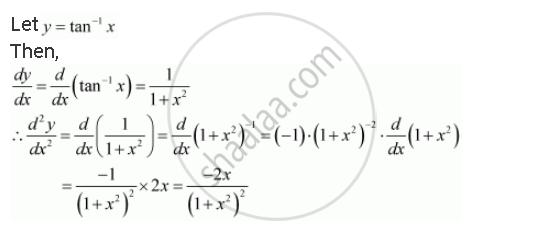Share

# Find the Second Order Derivatives of the Function. Tan–1 X - CBSE (Science) Class 12 - Mathematics

#### Question

Find the second order derivatives of the function.

tan–1 x

#### SolutionIs there an error in this question or solution?

#### APPEARS IN

NCERT Solution for Mathematics Textbook for Class 12 (2018 to Current)
Chapter 5: Continuity and Differentiability
Q: 8 | Page no. 183

#### Video TutorialsVIEW ALL 

Solution Find the Second Order Derivatives of the Function. Tan–1 X Concept: Second Order Derivative.
S